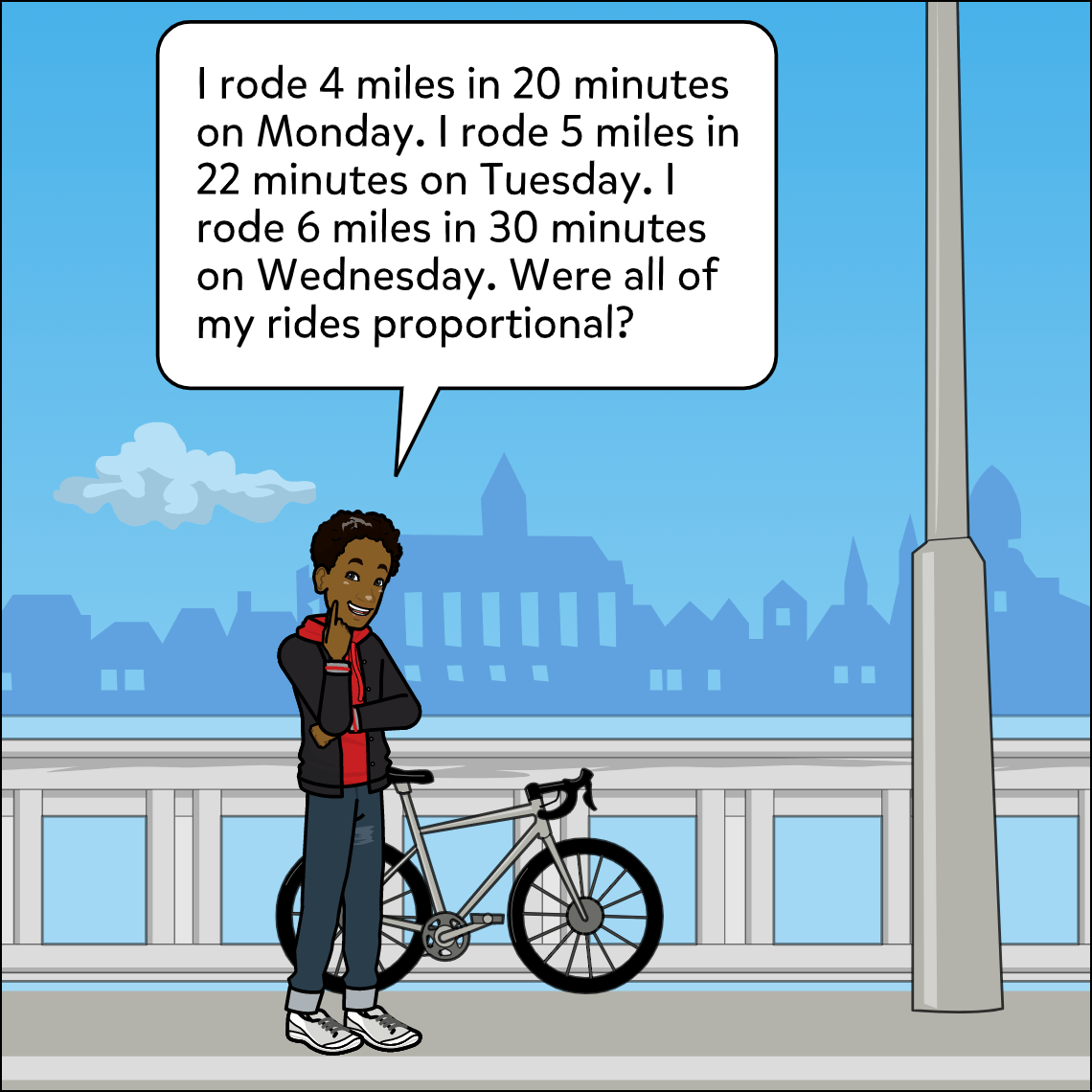Subject: Math

Lesson Length: 25 - 35 min

Topic: Ratios & Proportional Relationships- Proportional Relationships

Objective: Explanation

Standards: CCSS.MATH.CONTENT.7.RP.A.2. A,B,C

Brief Description: Analyze proportional relationships and use them to solve real-world and mathematical problems. Students will justify and explain if two given quantities are proportional.

Know Before You Start: Students should be familiar with proportional quantities prior to this lesson. This lesson could be used as a checkpoint or formative assessment to gauge student understanding of proportional quantities.Hook: Students should turn to a partner and explain how they know if two quantities are proportional. Share several responses to check for understanding.

Activity:

• Students will choose one of the sample comic panels to respond to.
• Students will use a caption at the top of their comic to denote which sample comic they are referring to (1 or 2).
• Students will create a 2-4 frame comic explaining whether the two quantities in the sample they chose are proportional or not.
• If they are proportional, students will explain and justify how they know.
• If they are not proportional, students will explain and justify how they know and provide a number that would make the relationship proportional.

Closure: As a class, students will decide which sample comic panel is proportional and which is not.

Resources:

• Students may need scrap paper to determine if the quantities are proportional before creating their comic.
• Example comic to print or display: Comic